Geometry

# Tangent and Secant Lines Warmup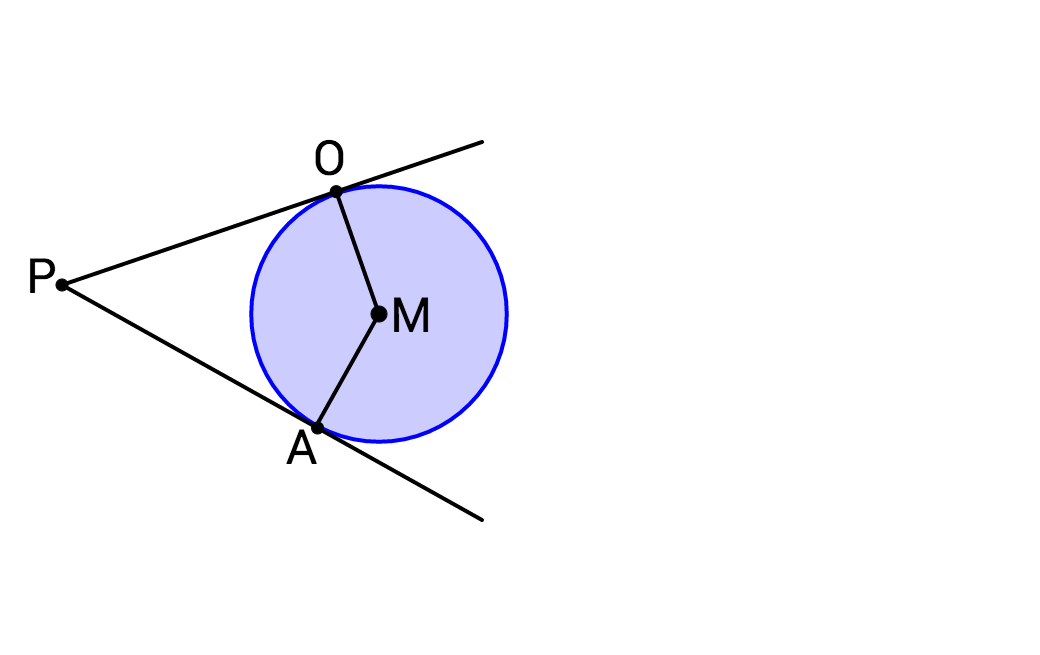Given the two lines tangent to circle $$M,$$ what is the measure of $$\angle POM + \angle PAM ?$$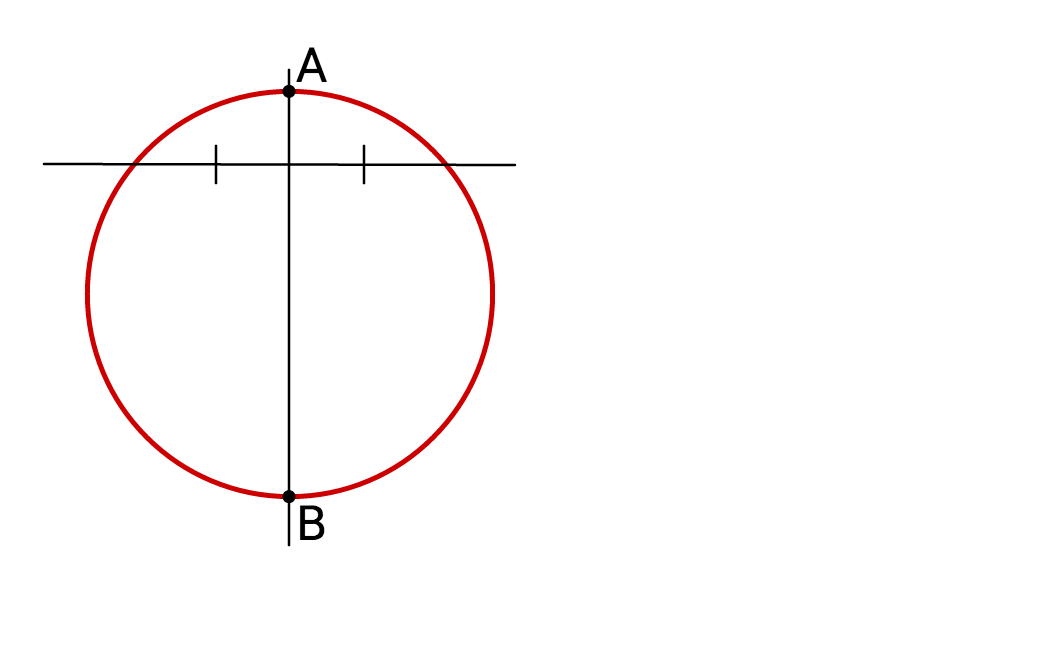The lines shown in the diagram are perpendicular.

True or False: Segment $$AB$$ must be the diameter of the circle.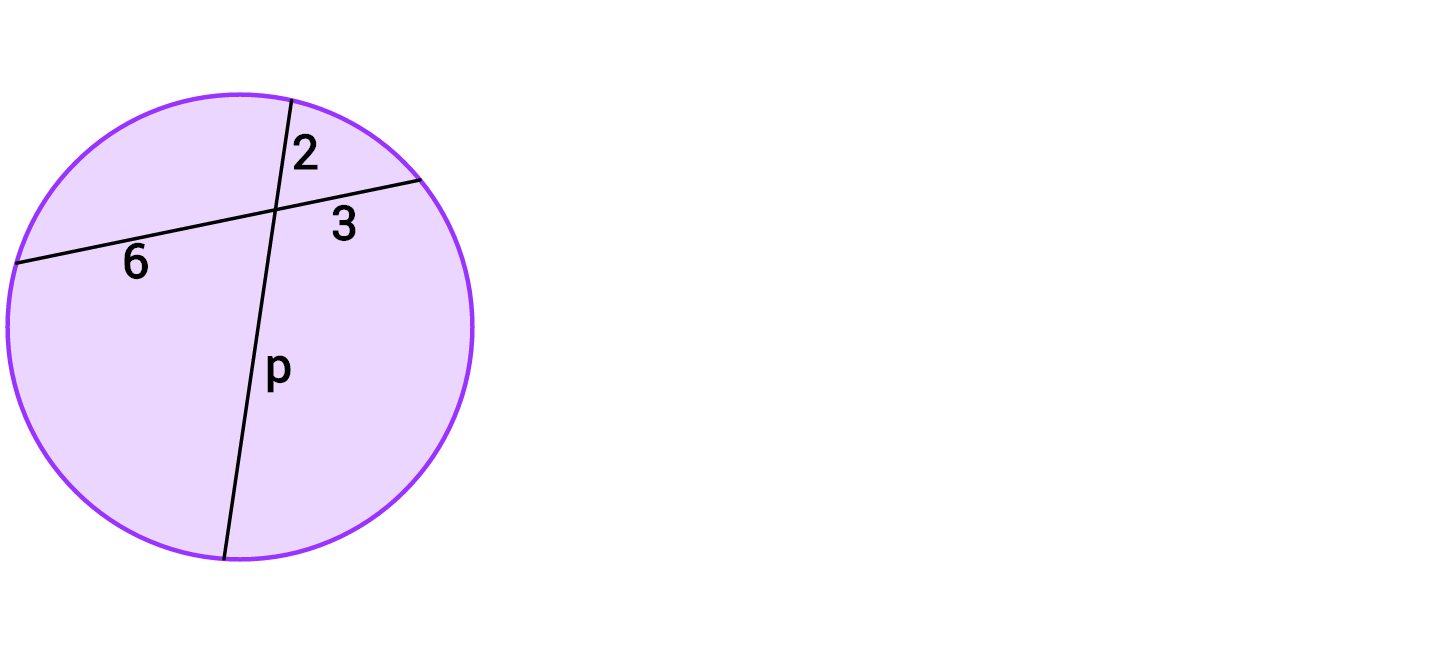What is the value of $$p?$$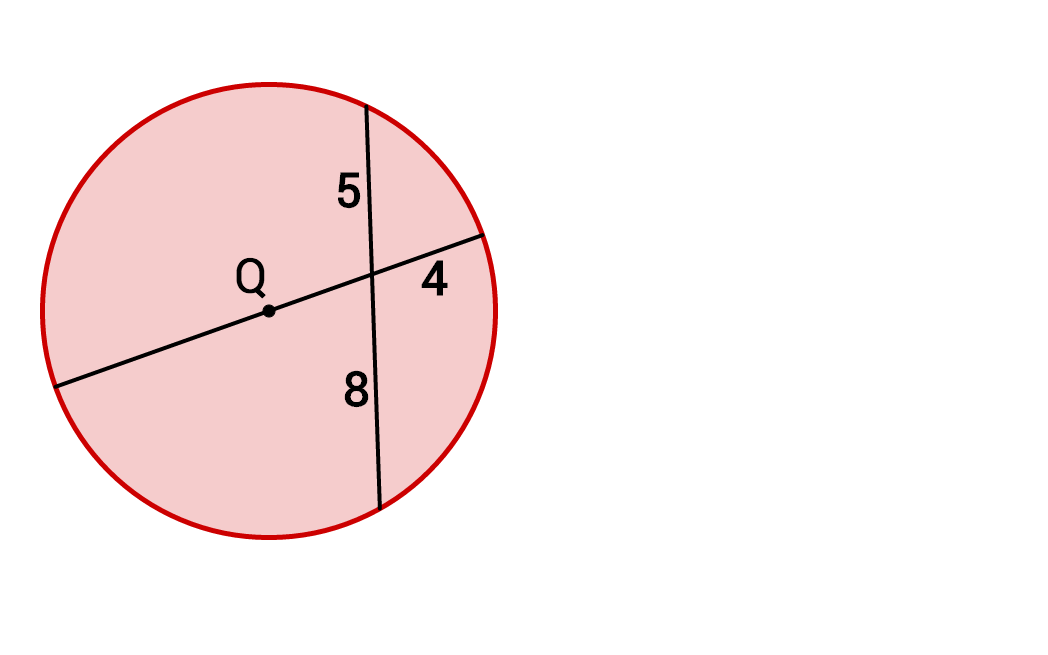What is the radius of circle $$Q?$$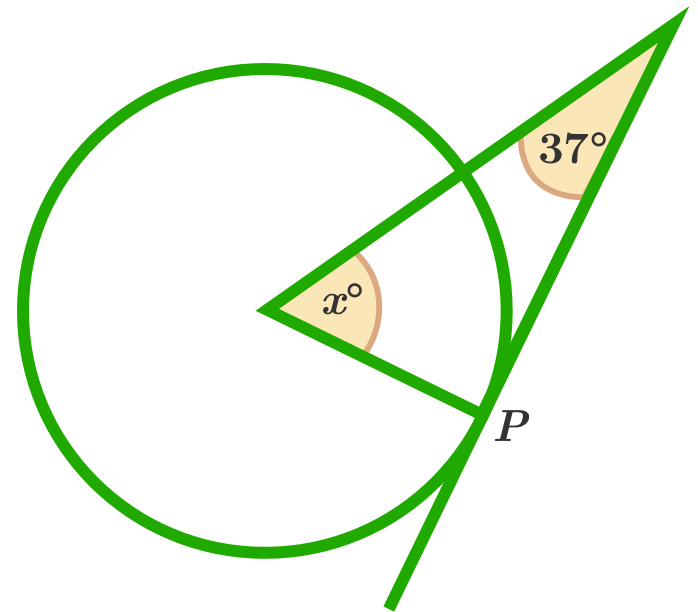If the line is tangent to the circle at point P and the vertex of the angle at $$x$$ is the center of the circle, what is the value of $$x?$$

×

Problem Loading...

Note Loading...

Set Loading...